#Interactive Real Analysis

Next | Previous | Glossary | Map

## 4.2. Convergence Tests

### Comparison Test

Suppose that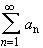converges absolutely, andis a sequence of numbers for which | bn || an | for all n > N. Then the seriesconverges absolutely as well.

If the seriesconverges to positive infinity, andis a sequence of numbers for which anbn for all n > N. Then the seriesalso diverges.

This is a useful test, but the limit comparison test, which is rather similar, is a much easier to use, and therefore more useful. However, this comparison test is very easy to memorize: Assuming that everything is positive, for simplicity, say we know that:
| b n || a n |
for all n. then just sum both sides to see what you get formally:
•• Then:
• If the left sides equals infinity, so must the right side.
• If the right side is finite, the left side also converges.
 Examples 4.2.4:Doesconverge or diverge ?Doesconverge or diverge ?
Proof:

The proof, at first glance, seems easy: Suppose thatconverges absolutely, and | b n || a n | for all n. For simplicity, assume that all terms in both sequences are positive. Let

S N =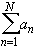and T N =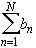Then we have that
T NSN
Since the left side is a convergent sequence, it is in particular bounded. Hence, the right side is also a bounded sequence of partial sums. Therefore it converges.

This proof wrong, because it does show that the sequence of partial sums is bounded but it is not necessarily true that a bounded series also converges - as we know.

However, this proof, slightly modified, does work: Again, assume that all terms in both sequences are positive. Sinceconverges, it satisfies the Cauchy criterion:

|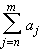| <if m > n > N. Since | b n || a n | we then have
|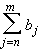||| <if m > n > N. Hencesatisfies the Cauchy criterion, and therefore converges.

The proof for divergence is similar.Next | Previous | Glossary | Map